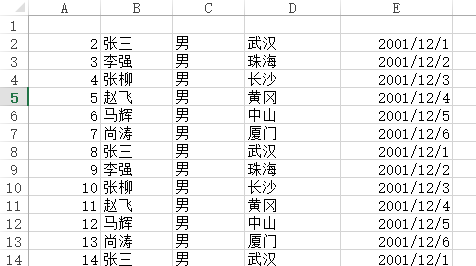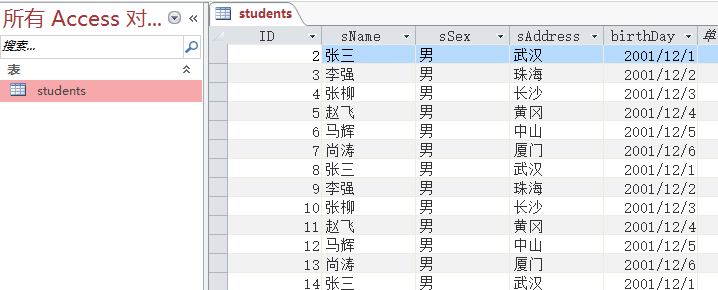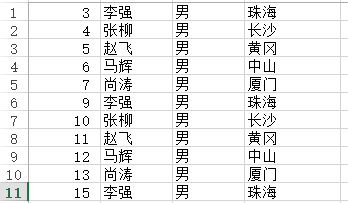• VBA获取access表名和各个表字段的数据类型
• Excel+vba通用SQL查询输出器源码 用于对Excel表格的SQL查询，被SQL操作的Excel表格必须有表头，作为类似数据库的字段，并位于表格第一行，SHEET1名称被指定时请注意替换默认的from后的表名称。
• 主要功能包含：一是VBA连接SQL Server数据库查询的功能，二是根据自动获取的日期动态将结果数据导出为多个Excel文档的功能。导出的文件命名为机构编码和日期动态命名方法，格式：机构编码+主文件名+日期，生成的...
• 多个工作簿，且每个工作簿中的工作表个数不定，字段不定，最终以指定字段生成一个新的工作簿。主要应用了数组和条件控制等。
• 2、excel表格保留一行标题行，并把第一列数据填写为拆分项（文件拆分时将第一列的内容进行归类合并为一个文件），整个表格不要合并单元格； 3、在打开的EXCEL工作表名称上点右键选择查看代码； 4、选择菜单栏：...
• 【例1】使用Connection对象，从数据库test.accdb的students表查询所有数据并存放到Sheet1表中。 Sub test()  Dim cnn, rst  Set cnn = CreateObject("ADODB.Connection")  Set rst = CreateObject...
【例1】使用Connection对象，从数据库test.accdb的students表查询所有数据并存放到Sheet1表中。
Sub test()
Dim cnn, rst
Dim conStr$, sqlStr$
conStr = "provider=Microsoft.ace.OLEDB.12.0;Data Source=" & ThisWorkbook.Path & "\test.accdb;"
cnn.Open conStr
sqlStr = "select * from students"
Worksheets("Sheet1").[A2].CopyFromRecordset cnn.Execute(sqlStr)
MsgBox "操作完成"
cnn.Close
End Sub
操作结果如下：【例2】使用RecordSet对象，从数据库test.accdb的students表查询所有数据并存放到Sheet1表中。

Sub test()
Dim cnn, rst
Dim conStr$, sqlStr$

conStr = "provider=Microsoft.ace.OLEDB.12.0;Data Source=" & ThisWorkbook.Path & "\test.accdb;"
cnn.Open conStr

sqlStr = "select * from students"
rst.Open sqlStr, cnn

Worksheets("Sheet1").[A2].CopyFromRecordset rst

rst.Close
cnn.Close
Set rst = Nothing
Set cnn = Nothing
End Sub
注：数据库及表均跟例1相同。
将读取的数据存储到数组
数据库记录如下：读取结果如下：Sub test()
Dim cnn, rst
Dim conStr$, sqlStr$
Dim arr(), title()

conStr = "provider=Microsoft.ace.OLEDB.12.0;Data Source=" & ThisWorkbook.Path & "\test.accdb;"
cnn.Open conStr

sqlStr = "select * from students"
title = Array("ID", "sName", "sSex", "sAddress")    '数据库中需要提取内容的字段(部分或者全部)
Worksheets("Sheet1").[A1].Resize(UBound(arr, 2) + 1, UBound(arr, 1) + 1) = Application.WorksheetFunction.Transpose(arr)

rst.Close
cnn.Close
Set rst = Nothing
Set cnn = Nothing
End Sub

注：getrows的第三个参数为需要读取的字段。第二个字段可以取值如下
常量  值  含义  adBookmarkCurrent  0  从当前记录开始  adBookmarkFirst  1  从第1条记录开始  adBookmarkLast  2  从最后一条记录开始 
展开全文Excel Access 数据库
• 'A1 B2 C3 D4 E5 F6 G7 H8 I9 J10 'K11 L12 M13 N14 O15 P16 Q17 R18 'S19 T20 U21 V22 W23 X24 Y25 Z26 Public Sub Connect() Dim KqData, i Dim result(1 To 10000, 1 To 2) KqData = Intersect(Acti...
'A1 B2 C3 D4 E5  F6 G7 H8 I9 J10
'K11 L12 M13 N14 O15 P16 Q17 R18
'S19 T20 U21 V22 W23 X24 Y25 Z26
Public Sub Connect()
Dim KqData, i
Dim result(1 To 10000, 1 To 2)
KqData = Intersect(ActiveSheet.UsedRange, ActiveSheet.Range("A:G"))
For i = 1 To UBound(KqData, 1)
result(i, 1) = "10" & Format(KqData(i, 2)) & Format(KqData(i, 3), "000")
Next i
ActiveSheet.[E1].Resize(UBound(KqData, 1), 1) = result
End Sub
测试表格内容
101张外网0202李外网1303张外网2404李外网3
展开全文• '子查询（嵌套查询） 'sql = "select 部门,count(*) as 人数 from (select * from 员工 where 年龄>=30) group by 部门" 'sql = "select 部门 as 所在部门,count(*) as 人数 from 员工 group by 部门 order by ...
Option Explicit
Sub chanxun()

Dim con As New ADODB.Connection  '声明并创建连接对象
Dim rs As New ADODB.Recordset    '声明并创建记录集对象
con.Open "provider=microsoft.ace.oledb.12.0;data source=" & ThisWorkbook.Path & "\学生管理.accdb"
Dim sql As String
'sql = "select * from 学生,课程,成绩 where 学生.学号=成绩.学号 and 课程.课程代码=成绩.课程代码"
'sql = "select 学生.学号,姓名,课程.课程代码,课程名称,成绩 from 学生,课程,成绩 where 学生.学号=成绩.学号 and 课程.课程代码=成绩.课程代码"
'sql = "select 课程名称,avg(成绩) as 平均成绩 from 课程,成绩 where 课程.课程代码=成绩.课程代码 group by 课程名称"
'内连接
'sql = "select 课程名称,avg(成绩) as 平均成绩 from 课程 inner join 成绩 on 课程.课程代码=成绩.课程代码 group by 课程名称 having avg(成绩)>=85"
'外连接
'sql = "select 姓名,性别,职称,院系.院系编号,院系名 from 导师 left join 院系 on 导师.院系编号=院系.院系编号"
'sql = "select 姓名,性别,职称,院系.院系编号,院系名 from 导师 right join 院系 on 导师.院系编号=院系.院系编号"
'sql = "select 姓名,性别,职称,院系.院系编号,院系名 from 导师 full outer join 院系 on 导师.院系编号=院系.院系编号"
'自连接
'sql = "select * from 员工 t1 inner join 员工 t2 on t1.姓名=t2.姓名"
'sql = "select distinct t1.编号,t1.姓名,t1.年龄,t1.部门 from 员工 t1 inner join 员工 t2 on t1.姓名=t2.姓名 where t1.编号<>t2.编号 order by t1.姓名"
'子查询（嵌套查询）
'sql = "select 部门,count(*) as 人数 from (select * from 员工 where 年龄>=30) group by 部门"
'sql = "select 部门 as 所在部门,count(*) as 人数 from 员工 group by 部门 order by 部门"
'提取出年龄比平均年龄大的员工信息
'sql = "select 姓名,性别,年龄,职务,部门 from 员工 where 年龄>(select avg(年龄) from 员工)"
'查询年龄排在5-10 名的员工信息
sql = "select top 6 姓名,性别,年龄,职务,部门 from 员工  where 年龄 not in( select top 4 年龄 from 员工 order by 年龄)"
Set rs = con.Execute(sql)
Dim i As Integer
For i = 0 To rs.Fields.Count - 1
Cells(1, i + 1) = rs.Fields(i).Name
Next
Range("A2").CopyFromRecordset rs
Columns.AutoFit
rs.Close: Set rs = Nothing
con.Close: Set con = Nothing
End Sub

可以试着改一下，试着查询
展开全文• 自制VBA多列相同字段匹配程序.从数据源匹配取数的问题，写了个小的VBA程序。请参考和指正！o(∩_∩)o...
• 支持多有素匹配（1：大小写转换，2：全角半角转换，3：切换新sheet）
• 首先，将需要拆分的sheet命名为“明细”，接下来运行此代码，提示操作即可。 在这里插入代码片 Sub chaifen() '定义变量类型 Dim sht, sh1, sh2 As Worksheet Dim k, i, j As Integer Dim irow As Integer Dim col...
首先，将需要拆分的sheet命名为“明细”，接下来运行此代码，按提示操作即可。
在这里插入代码片
Sub chaifen()
'定义变量类型
Dim sht, sh1, sh2 As Worksheet
Dim k, i, j As Integer
Dim irow As Integer
Dim col As Integer
Dim str As String

'程序开始是要求输入按哪一列拆分数据
col = InputBox("请输入你要按哪一列拆分数据")

'获取所选择的文件夹路径
Set fileDlg = Application.FileDialog(msoFileDialogFolderPicker)

With fileDlg

If .Show = -1 Then

For Each fld In .SelectedItems

str = fld

Next fld

End If

End With

Application.ScreenUpdating = False '防止屏幕一直闪动

'开始时先删除无意义的表，只留下需要拆分的sheet

If Sheets.Count > 1 Then
For Each sht1 In Sheets
If sht1.Name <> "明细" Then
sht1.Delete
End If
Next
End If

'拆分明细这张sheet
irow = Sheet1.Range("a1048576").End(xlUp).Row '用于计算sheet1一共有几行
For i = 2 To irow
k = 0
For Each sht In Sheets
If sht.Name = Sheet1.Cells(i, col) Then
k = 1
End If
Next

If k = 0 Then
Sheets(Sheets.Count).Name = Sheet1.Cells(i, col)
End If
Next
'拷贝数据到“明细”后面的sheet2,sheet3,sheet4....中
For j = 2 To Sheets.Count
Sheet1.Range("a1:s" & irow).AutoFilter Field:=col, Criteria1:=Sheets(j).Name
Sheet1.Range("a1:s" & irow).Copy Sheets(j).Range("a1")
Next

Sheet1.Range("a1:s" & irow).AutoFilter '取消筛选
Sheet1.Select

'将其中的sheets拆分为多个Excel文件

For Each sht2 In Sheets
If sht2.Name <> "明细" Then
sht2.Copy
ActiveWorkbook.SaveAs Filename:=str & "\" & sht2.Name & ".xlsx"
ActiveWorkbook.Close
End If
Next

Application.ScreenUpdating = True
MsgBox "已处理完毕"

End Sub


展开全文• 字段名提取 用Collection对象方法： Private Sub UIButtonFields_Click() ' Part 1: Get the feature class and its fields. Dim pMxDoc As IMxDocument Dim pMap As IMap Dim pFeatureLayer As IFeeach list class 框架
• excel 透视表 vba 使用Excel VBA删除数据透视表计算字段 (Remove Pivot Table Calculated Field With Excel VBA)Yesterday, I started out with the best of intentions, planning to get some work done, and find ...java python mysql 大数据 人工智能
• VBA给EXCEL表格做排序列名称或是标题给表格排序 列名称或是标题给表格排序 在VBA编程时，经常会对表格内容进行排序，为了方便对不同需求下的内容排序，编制了下面这个函数，此函数可以根据给定的列名称（A、...
• 在ArcGIS属性表中，一般不会包含实体几何信息，可以采取VBA进行计算。下面是几个简单的代码:1推荐给不会使用AO的朋友;2可以保存为CAL文件以备下次方便使用
• ## Excel VBA 多条件查询

万次阅读 2013-08-26 15:19:12
用以下两个公式都可以实现多条件查询，同样也可以用VBA代码来实现 函数公式1： '=INDEX(Sheet3!$F$2:$F$20,MATCH(B2&C2&D2&E2,Sheet3!$B$2:$B$20&Sheet3!$C$2:$C$20&Sheet3!$D$2:$D$20&Sheet3!$E$2:$E$20,0)) 函数...
• ' 依条件设置查询数组，返回包含查询字段（或全部字段）的数组，可多条件组合。 ' 条件运算符包括：> = < >= <= <> , like(正则表达式) ' '附注： ' 使用此函数，需要在文件中引用正则表达式脚本 Microsoft VBScript...
• 一个企业里常用的vba工具，可以将一个表的数据，自定义要抽取的数据字段，抽取到另一个表中。 包含演示数据 不用修改代码即可使用，超好用！
•excel Access sql
• 看似没用的东西，在真正需要的时候就...'Union (AlL) 多个select查询结果合并在一起 Sub 合并工作表数据() Dim data As New 类1 Dim sql As String sql = "select * from [Sheet1$a:c] union all select * fr... • 主要介绍了分组字符合并SQL语句 字段合并字符串之一(简单合并),需要的朋友可以参考下字符合并 • Sub 分类() Dim A Dim cnn, SQL$ Set cnn = CreateObject("adodb.connection") Set RS = CreateObject("adodb.recordset") cnn.Open "Provider = Microsoft.ace.Oledb.12.0;Extended Properties =TEXT;...
• mr = .MaxRecords 'default 0,now 100,设置或返回从一个查询返回 Recordset 对象的的最大记录数目。 pc = .PageCount 'default -1,返回一个 Recordset 对象中的数据页数。 ps = .PageSize 'default 10,设置或返回 ...
• 作为引子，先发一篇VBA按条件拆分工作表的方法，希望有所帮助。 作为例子，创建一个名为“ALL”的工作表，存放了13位不同年龄、不同部门的员工名单。字段包括员工号/姓名/部门和年龄。 现要求按照部门，将十三位员工...拆分表格 工作效率 数据 excel
• ## VBA_输出SQL查询数据

千次阅读 2017-04-22 22:21:44
'循环查询到的列名，把列名的数量定义循环。 rs.Open strSql, cn '执行strSQL所含的SQL命令，结果保存在rs记录集对象中 i = 0 Lm = rs.Fields.Count '列名的数量定义 While i < Lm  sht.Cells(2, i +...
• select * from Pub_sssEmpxx where (Code like'%100%') or (DID like'%"100011"%') or ([Eame] like'%" & zipcode_key & "%') order by ode 　 转载于:https://www.cnblogs.com/914556495wxkj/p/7111...
• Set Rst = Conn.Execute(strSQL) '执行查询，并将结果输出到记录集对象 With Sheet1 .Cells.Clear For i = 0 To Rst.Fields.Count - 1 '填写标题 .Cells(1, i + 1) = Rst.Fields(i).Name Next i .Range("A2")...
• 功能：可以将一个EXCEL文档，指定字段，拆分成多个EXCEL文件，生成的文件名， 允许加前缀和后缀，并保留母表格式。...